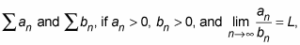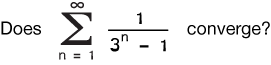# Comparison Test for Convergence: Limit / Direct

There are three tests in calculus called a “comparison test.” Both the Limit Comparison Test (LCT) and the Direct Comparison Test(DCT) determine whether a series converges or diverges. A third test is very similar and is used to compare improper integrals.

Contents :

## Limit Comparison Test (Limit Test for Convergence / Divergence)

The Limit Comparison Test (LCT) is used to find out if an infinite series of numbers converges (settles on a certain number) or diverges.

The LCT is a relatively simple way to compare the limit of one series with that of a known series.  This is accomplished by taking a known convergent or divergent series of numbers and multiplying each by another number (x).

• A series converges if a limit exists (i.e. it converges to a finite value).
• A divergent series will not have a limit; The partial sums (sums of part of the sequence) either have no limit or they approach infinity.

The value of x can be either large or small, since any number times the finite sum of the original series will be a finite number. The series terms will always be positive when working with the Limit Comparison Test.A caution when using the Limit Comparison Test:  While the smaller of two series may converge that does not tell you anything about the larger series.  You may very well have a situation where the smaller series converges while the larger series is divergent.

## Limit Comparison Test: ExampleStep 1: Arrange the limit.

Step 2: Multiply by the reciprocal of the denominator.Step 3: Divide every term of the equation by 3n.Dividing by 3n we are left with:To evaluate this equation, first notice that n → ∞.  The fraction above is equal to 1 which is greater than zero.  Since the amount is greater than zero we know that through the limit comparison test that the series converges with a known convergent series.

## Direct Comparison Test

The Direct Comparison Test is sometimes called just a comparison test, but shortening the name can cause confusion with the LCT. The DCT is formally stated as follows:

Let 0 < an ≤ bn for all n. Then:Let’s say you have two series, both comprised of positive terms. One of the series has terms that are always greater than the other series. If the larger series is convergent then the smaller is convergent. Similarly, if the smaller series is divergent then the larger series is divergent.

The comparison test only applies if the terms are smaller than the terms of a convergent series bn or larger than those of a divergent series an. Also, in order for the test to work, both series have to start in the same place.

## Cautions in Using the DCT

The test has a couple of pitfalls, so be careful when using it.

First, the test only tells you what happens to the smaller of the two series. Be careful in applying the test: don’t make the mistake of switching the two series, because a convergent or divergent smaller series tells you nothing about the behavior of the larger series.

You could modify the test a little to ignore the first few values of n, up to a certain number n. For example, you could essentially ignore the first dozen inputs (you can do this because convergence isn’t dependent on the first few terms). How many terms you choose to ignore depends on the series, so it can be a little tricky to try and modify the test if you aren’t very knowledgeable about series.

## Direct Comparison Test Example

Take the following series:Sidebar: Something that should jump out, if you have a good grasp of different series and algebra, is that 1/n3 is a convergent p series.

The 4 that I pulled out in front is irrelevant for this problem, because 4 * a convergent series is still going to be convergent. I also deleted the “4” in the denominator here, because 4 is tiny compared to n3. This was only done to show you the closeness to the p series.

Back to the problem at hand:

When you pull the 4 out front, you’re left with 1 / n3 + 4. To use the DCT, you just have to show that your series is less than 1 / n3. The larger denominator means that the series 1 / n3 + 4will always be less than 1 / n3, so the series is convergent.

## Which one do I use?

Part of the problem of having so many tests for convergence and divergence is knowing which one to use. This is particularly true of the Direct Comparison Test and Limit Comparison Test, which are often confused.

There isn’t an easy answer to the question which one do I use? What you have to do is to look at the series and see if you can first make a direct comparison (which is probably the easier of the two tests).

If you can’t use the DCT, then use the LCT.

## Comparison Test for Improper Integrals

The Comparison Test for Improper Integrals is a rule to tell the difference between two convergent and divergent improper integrals.

Let’s call the two continuous functions we’re comparing “f(x)” and “g(x)”, where and g(x) is smaller than f(x). The relatively simple rule allows us to make discuss convergence or divergence without actually evaluating some integrals.

## Formal Definition of The Comparison Test for Improper Integrals

If f(x) and g(x) are continuous on the interval [1, ∞) and 0 ≤ f(x) < g(x) for all x ≥ a, then:## Example

The following integral is difficult to integrate:However, we can compare it to a much simpler integralValues for e-x2 will always be smaller than e-x (if you aren’t sure about this, plug a few values for e-x2 and e-x into a calculator) so we can set up an inequality:
e-x2 ≤ e-x.
The function e-x is convergent. Therefore, we can use the first part of the rule and conclude that e-x2 is also convergent .

## References

 Larson, R. & Edwards, B. (2009). Calculus. Brooks/Cole; 9 edition.
 Taneja, H. (2010). Advanced Engineering Mathematics. I K International Publishing House.

CITE THIS AS:
Stephanie Glen. "Comparison Test for Convergence: Limit / Direct" From StatisticsHowTo.com: Elementary Statistics for the rest of us! https://www.statisticshowto.com/sequence-and-series/series-convergence-tests/comparison-test-limit-direct/